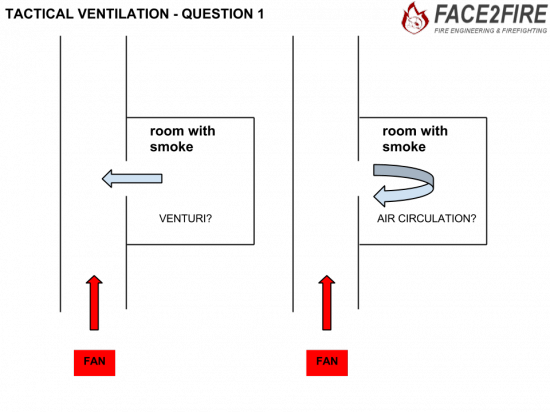# Venturi or air circulation?, that’s the question.

Sometimes firefighters have to use vpp techniques to empty a room of smoke. But the questions is…with whome physical principles they can do this task possible?## Venturi effect

The Venturi effect is the reduction in fluid pressure that results when a fluid flows through a constricted section of pipe. The Venturi effect is named after Giovanni Battista Venturi (1746–1822), an Italian physicist.## Background

The Venturi effect is a jet effect; as with a funnel the velocity of the fluid increases as the cross sectional area decreases, with the static pressurecorrespondingly decreasing. According to the laws governing fluid dynamics, a fluid’s velocity must increase as it passes through a constriction to satisfy the principle of continuity, while its pressure must decrease to satisfy the principle of conservation of mechanical energy. Thus any gain inkinetic energy a fluid may accrue due to its increased velocity through a constriction is negated by a drop in pressure. An equation for the drop in pressure due to the Venturi effect may be derived from a combination of Bernoulli’s principle and the continuity equation.

The limiting case of the Venturi effect is when a fluid reaches the state of choked flow, where the fluid velocity approaches the local speed of sound. In choked flow the mass flow rate will not increase with a further decrease in the downstream pressure environment.

However, mass flow rate for a compressible fluid can increase with increased upstream pressure, which will increase the density of the fluid through the constriction (though the velocity will remain constant). This is the principle of operation of a de Laval nozzle. Increasing source temperature will also increase the local sonic velocity, thus allowing for increased mass flow rate.

Referring to the diagram to the right, using Bernoulli’s equation in the special case of incompressible flows (such as the flow of water or other liquid, or low speed flow of gas), the theoretical pressure drop at the constriction is given by:whereis the density of the fluid,is the (slower) fluid velocity where the pipe is wider,is the (faster) fluid velocity where the pipe is narrower (as seen in the figure). This assumes the flowing fluid (or other substance) is not significantly compressible – even though pressure varies, the density is assumed to remain approximately constant.

## Examples

The Venturi effect may be observed or used in the following:

The Bernoulli Principle and its corollary, the Venturi effect, are essential to aerodynamic as well as hydrodynamic design concepts. Airfoil and hydrofoil designs to lift and steer air and water vessels (airplanes, ships and submarines) are derived from applications of the Bernouoli Principle and the Venturi effect, as are the instruments that measure rate of movement through the air or water (velocity indicators). Stability indication and control mechanisms such as gyroscopic attitude indicators and fuel metering devices, such as carburetors, function as a result of gas or fluid pressure differentials that create suction as demonstrated and measurable by gas/fluid pressure and velocity equations derived from the Bernoulli Principle and the Venturi Effect.

A simple way to demonstrate the Venturi effect is to squeeze and release a flexible hose in which fluid is flowing: the partial vacuum produced in the constriction is sufficient to keep the hose collapsed.

Venturi tubes are also used to measure the speed of a fluid, by measuring pressure changes at different segments of the device. Placing a liquid in a U-shaped tube and connecting the ends of the tubes to both ends of a Venturi is all that is needed. When the fluid flows though the Venturi the pressure in the two ends of the tube will differ, forcing the liquid to the «low pressure» side. The amount of that move can be calibrated to the speed of the fluid flow.

Sources: Wikipedia